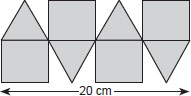# User Forum

Subject :IMO    Class : Class 6

Priya bent a plastic wire to form the given figure. The figure is made up of 4 squares and 4 equilateral triangles. Find the length of wire.A 110 cm
B 90 cm
C 100 cm
D 80 cm

4 squares and 4 equilateral triangles means total 24 lines ie one square and equilateral triangle is made of 6 wires as per the diagram 5 x 24 so the answer will be 120, If 100 is the answer they must not highlighted the center line..

## Ans 1:

Class : Class 6
i also think answer should be 120. this answer is given with not counting the middle line

## Ans 2:

Class : Class 6
But the plastic wire is bent in the middle too, as shown in the figure. But the middle line is not considered? The answer should be 120, not 100. Its coming for me by including the middle line. Please define it clearly. Requested

## Ans 3:

Class : Class 6
the answer is c = 100 because the middle line is not counted .

## Ans 4:

Class : Class 7
I too have this doubt

Class : Class 6

## Ans 6:

Class : Class 6
The middle line should not be there.Without the middle line the answer is C=100

## Ans 7:

Class : Class 6
I think the answer is A =110

## Ans 8:

Class : Class 7
The question must have been made a little clearer. If the correct answer is 100, then they might be asking for the perimeter of the above figure.

Class : Class 6
same doubt!

Class : Class 6
Same doubt

## Ans 11:

Class : Class 7
I think the answer should be 120. Could anyone explain how it is 100?